# 基于改进遗传算法的无人机搜索路径规划的研究Research on UAV Search Path Planning Based on Improved Genetic Algorithm

DOI: 10.12677/ORF.2020.103021, PDF, HTML, XML, 下载: 90  浏览: 336  国家自然科学基金支持

Abstract: Aiming at the problem of UAV searching for the target with uncertain moving speed and direction, this paper designs a search path planning algorithm based on improved genetic algorithm. The traditional method based on analysis has not strong adaptability and is not convenient for the realization of computer automatic calculation. In this paper, a search path planning algorithm based on improved genetic algorithm is proposed, which considers the probability time and other parameters of the target moving direction as a dynamic artificial potential field, improves the adaptability of the algorithm for solving the search path planning problem, and has a high degree of adaptability and automation for the complex search area and the target moving probability. According to the idea of survival of the fittest in genetic algorithm, a more optimal search path is selected. In this process, the path point of UAV is regarded as the gene of organism; the search path is regarded as the chromosome of organism; and then the search path is optimized through hybridization, selection, mutation and other operations. Then, an example is set up for simulation calculation. From the simulation results, we can see that the search path planning algorithm based on the improved genetic algorithm is feasible, and has the advantages of flexibility, efficiency and automation.

1. 引言

2. 目标分布概率模型

$x=vt\mathrm{cos}\theta$$y=vt\mathrm{sin}\theta$ 时：

$f\left(x,y,t\right)=\frac{p\left(\theta \right)}{{v}_{\mathrm{max}}-{v}_{\mathrm{min}}}$ (2.1)

$f\left(x,y,t\right)=0$ (2.2)

3. 无人机探测模型建模

$MK=h/\mathrm{tan}\frac{\alpha }{2}$ (3.1)

$OK=h/\mathrm{sin}\frac{\alpha }{2}$ (3.2)

$PQ=20K\mathrm{tan}\frac{\lambda }{2}$, $S=P{Q}^{2}$ (3.3)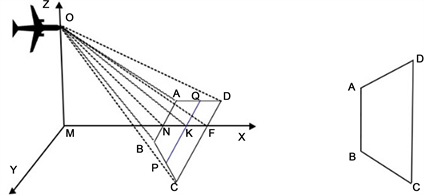x、y、z轴的单位是m，用来表示无人机飞行时的位置关系。

Figure 1. Ground projection diagram of UAV detection field of view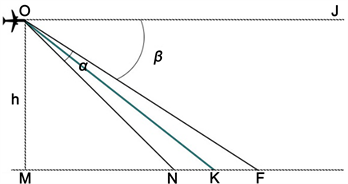x、y、z轴的单位是m。

Figure 2. View angle range diagram of UAV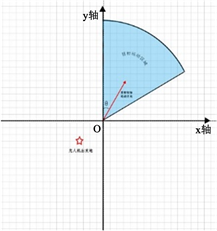x、y轴的单位是km。下同。

Figure 3. Grid diagram

4. 算法设计

4.1. 路径编码

4.2. 交叉算子

${p}_{1}=\left[{g}_{1},{g}_{2},{g}_{3},\cdots ,{g}_{k-1},{g}_{k},{g}_{k+1},\cdots ,{g}_{m}\right]$

${p}_{2}=\left[{x}_{1},{x}_{2},{x}_{3},\cdots ,{x}_{k-1},{x}_{k},{x}_{k+1},\cdots ,{x}_{m}\right]$

${p}_{3}=\left[{g}_{1},{g}_{2},{g}_{3},\cdots ,{g}_{k-1},{y}_{1},{y}_{2},\cdots ,{y}_{n},{x}_{k},{x}_{k+1},\cdots ,{x}_{m}\right]$

${p}_{4}=\left[{x}_{1},{x}_{2},{x}_{3},\cdots ,{x}_{k-1},{z}_{1},{z}_{2},\cdots ,{z}_{l},{g}_{k},{g}_{k+1},\cdots ,{g}_{m}\right]$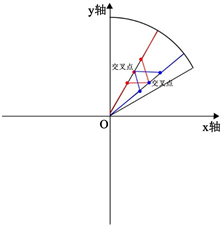Figure 4. Schematic diagram of cross operation

4.3. 变异算子

${p}_{1}=\left[{g}_{1},{g}_{2},{g}_{3},\cdots ,{g}_{k-1},{g}_{k},{g}_{k+1},\cdots ,{g}_{m}\right]$

${p}_{2}=\left[{g}_{1},{g}_{2},{g}_{3},\cdots ,{g}_{k-1},{B}_{1},{B}_{2},\cdots ,{B}_{n-1},{B}_{n},{B}_{n+1},\cdots ,{B}_{k},{g}_{k},{g}_{k+1},\cdots ,{g}_{m}\right]$

${p}_{3}=\left[{g}_{1},{g}_{2},{g}_{3},\cdots ,{g}_{k-1},{A}_{1},{A}_{2},\cdots ,{A}_{n-1},{A}_{n},{g}_{k+1},\cdots ,{g}_{m}\right]$

${p}_{4}=\left[{g}_{1},{g}_{2},{g}_{3},\cdots ,{g}_{k-1},{C}_{1},{C}_{2},\cdots ,{C}_{n-1},{C}_{n},{C}_{n+1},\cdots ,{C}_{k},{g}_{k+1},\cdots ,{g}_{m}\right]$

${B}_{n}$ 为变异后在 ${g}_{k-1},{g}_{k}$ 两个点之间插入的点， ${B}_{1},{B}_{2},\cdots ,{B}_{n-1}$${g}_{k-1}$${B}_{n}$ 点之间直线连接经过的网格， ${B}_{n+1},\cdots ,{B}_{k}$${B}_{n}$${g}_{k}$ 之间直线连接时经过的网格。

${p}_{3}$ 是删掉了点 ${g}_{k}$ 后得到的， ${A}_{1},{A}_{2},\cdots ,{A}_{n-1},{A}_{n}$${g}_{k-1}$${g}_{k+1}$ 之间直线连接时经过的网格。

${p}_{4}$ 是在删掉了一个点 ${g}_{k}$ 同时增加了一个点 ${C}_{n}$ 后得到， ${C}_{n}$ 为变异后在 ${g}_{k-1},{g}_{k+1}$ 两个点之间插入的点， ${C}_{1},{C}_{2},\cdots ,{C}_{n-1}$${g}_{k-1}$${C}_{n}$ 点之间直线连接时经过的网格， ${C}_{n+1},\cdots ,{C}_{k}$${C}_{n}$${g}_{k+1}$ 之间直线连接时经过的网格。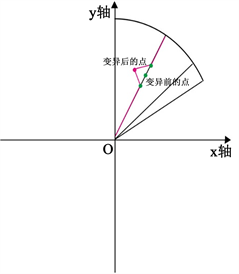Figure 5. Schematic diagram of mutation operation

4.4. 适应度函数

$F={\sum }_{i=1}^{m}f\left({x}_{i},{y}_{i},{t}_{i}\right)$ (4.1)

5. 算例Table 1. Probability table of possible target heading distribution

$f\left(x,y,t\right)=\left\{\begin{array}{l}f\left(v\mathrm{csc}{\theta }_{1},v\mathrm{sin}{\theta }_{1},t\right)=\frac{1}{4}\\ f\left(vt\mathrm{csc}{\theta }_{2},v\text{ }\text{tin}{\theta }_{2},t\right)=\frac{1}{4}\\ f\left(v\mathrm{csc}{\theta }_{3},v\text{ }\text{tin}{\theta }_{3},t\right)=\frac{1}{4}\\ f\left(v\mathrm{csc}{\theta }_{4},v\mathrm{sin}{\theta }_{4},t\right)=\frac{1}{4}\end{array}$ (5.1)

${x}_{i}=vt\mathrm{cos}\theta ,\text{\hspace{0.17em}}\text{\hspace{0.17em}}{y}_{i}=vt\mathrm{sin}\theta \text{\hspace{0.17em}}\text{\hspace{0.17em}}\left(i=1,2,3,\cdots ,m\right)$

$f\left(x,y,t\right)=\left\{\begin{array}{l}\frac{1}{8\Delta v}\text{\hspace{0.17em}}\text{\hspace{0.17em}}\text{\hspace{0.17em}}\text{\hspace{0.17em}}x\in {x}_{i},y\in {y}_{i}\\ 0\text{\hspace{0.17em}}\text{\hspace{0.17em}}\text{\hspace{0.17em}}\text{\hspace{0.17em}}\text{\hspace{0.17em}}\text{\hspace{0.17em}}\text{\hspace{0.17em}}\text{\hspace{0.17em}}\text{\hspace{0.17em}}\text{\hspace{0.17em}}\text{\hspace{0.17em}}\text{\hspace{0.17em}}否则\end{array}$ (5.2)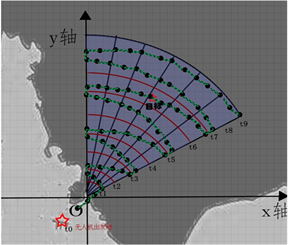Figure 6. A schematic of the calculated UAV’s search path

6. 总结展望

  周延安, 梅刚. 反辐射无人机搜索路径规划研究[J]. 舰船电子对抗, 2006(5): 8-10.  侯岳奇, 梁晓龙, 何吕龙, 刘流. 未知环境下无人机集群协同区域搜索算法[J]. 北京航空航天大学学报, 2019(2): 347-356.  李松, 刘海颖, 陈志明. 多无人机协同目标搜索路径规划仿真[J]. 计算机仿真, 2018(6): 46-50.  韩旭, 盛怀洁, 陈明建. 基于D-S证据理论的防辐射无人机群协同搜索[J]. 探测与控制学报, 2018(1): 80-87.  朱利, 符小卫. 基于Voronoi图质心的多无人机协同区域搜索算法[J]. 无人系统技术, 2019(2): 39-51.